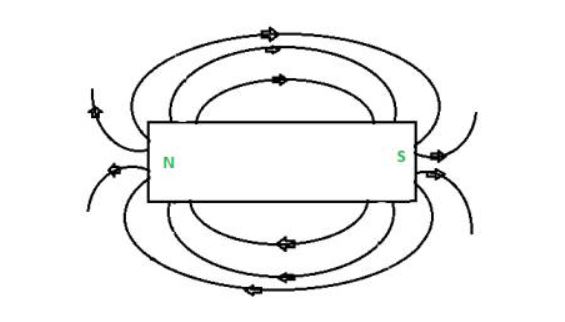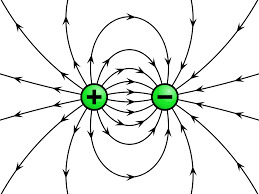Difference between Electric Field and Magnetic Field

• Difficulty Level : Expert
• Last Updated : 14 Jul, 2021

Electromagnetic field, a property of space caused by the motion of an electric charge. A stationary charge will produce only an electric field in the surrounding space. If the charge is moving, a magnetic field is also produced. An electric field can be produced also by a changing magnetic field. Electric and magnetic fields are both components of an electromagnetic field.

These two components occupy different planes relative to the cause of the electromagnetic field, for example, a moving electrical charge. This, and whether the charge generating the field is stationary or in motion, are the only differences.

Magnetic Field

The region around the magnetic where its poles exhibit a force of attraction or repulsion is called a magnetic field. The magnetic field also induces when the electric charges are moving in space or an electrical conductor.

Formula of Magnetic field is:

Magnetic force (B) = μ0/2πr

B = refers to the magnetic field magnitude in Tesla (T)

μ0 = refers to the permeability of free space (4π×10−7T⋅m/A)

I = refers to the magnitude of the electric current in amperes (A)

r = refers to the distance in meters (m)

When current is passed through a magnetic material, a magnetic field is produced, if a needle is placed near the material, on providing the electric charge (moving charges), the needle will deflect. The area to the point where the force is exerted by the magnetic material is called a magnetic field

Magnetic-Field Line

The moving charge carrier and magnet produce magnetic flux lines, and these lines are called magnetic field lines. It is a vector quantity because it has both magnitude and direction. The symbol B expresses the magnetic field, and it is measured in Tesla or Newton per meter.

Magnetic field = [M]1[T]2[I]-1Question 1: What is meant by a magnetic field?

Solution:

The region around the magnetic where its poles exhibit a force of attraction or repulsion is called a magnetic field. The magnetic field also induces when the electric charges are moving in space or an electrical conductor.

Question 2: Find the magnitude of the magnetic field that is 0.10 m away from a wire carrying a 3.00 A current? Also, the current has a vector direction out of the page (or screen), then what is the direction of the magnetic field?

Solution:

By using the formula we calculate the magnetic field:

B = μ02πr

B = (4π×10−7T⋅m/A)(3.00A)2π(0.10m)

B = (4π×10−7T⋅m/A)(3.00A)2π(0.10m)

B = (4π×10−7)(3.00)2π(0.10)

B = (4π×10−7)(3.00)2π(0.10)T

B = 4π(3.00)2π(0.10)×10−7 T

B = 2(30.0)×10−7 T

B = 60.0 ×10−7 T

B= 60.0 ×101−7 T

B = 60.0 ×10−6 T

B = 6.00 μT

Electric Field

The force around the electrical charge particle is called an electrical field or electric field intensity. In other words, it is an area around the electric field where the force line exists. It has magnitude and direction. Hence, it is a vector quantity. The symbol E expresses the electric field, and it is measured in newton/coulomb.Formula of Electric field = Force/ChargeQuestion 1: What do you understand by the term Electric Field?

The force around the electrical charge particle is called an electrical field or electric field intensity. In other words, it is an area around the electric field where the force line exists. It has magnitude and direction. Hence it is a vector quantity. The symbol E expresses the electric field, and it is measured in newton/coulomb.

Question 2: A force of 2 N is acting on the charge 6 μ C at any point. Determine the electric field intensity at that point.

Solution:

Given Force F = 2 N

Charge q = 6 μ C

Electric field formula is given by

E = F / q

= 2/6 × 10-6

Difference between Electric Field and Magnetic Field

My Personal Notes arrow_drop_up